Tips & Tricks

# Striving For Better C++ Code, Part I: Data Flow Analysis Basics

CLion comes with a built-in data flow analyzer, which runs constantly when you are writing your code and helps improve your code’s quality. It can reveal various code problems that might later lead to runtime issues, security breaches, and other vulnerabilities. Examples of these useful checks are checks for constant conditions, dead code, null pointer dereferences, memory leaks, and array index issues. We’re starting a series of blog posts to explain how some of these inspections work in CLion.

Today, we’ll look at the basics of data flow analysis, including how it works in general, while presenting several real-world examples where it can help you write better code.

## Control Flow Graph

All data flow inspections rely on the control-flow graph. This is a graph on which vertices are the statements in the program and edges are the control flow jumps between these statements (direct code execution, conditional jumps, loops, breaks, gotos, etc.).

For example, the control-flow graph at the right represents the function `foo` on the left: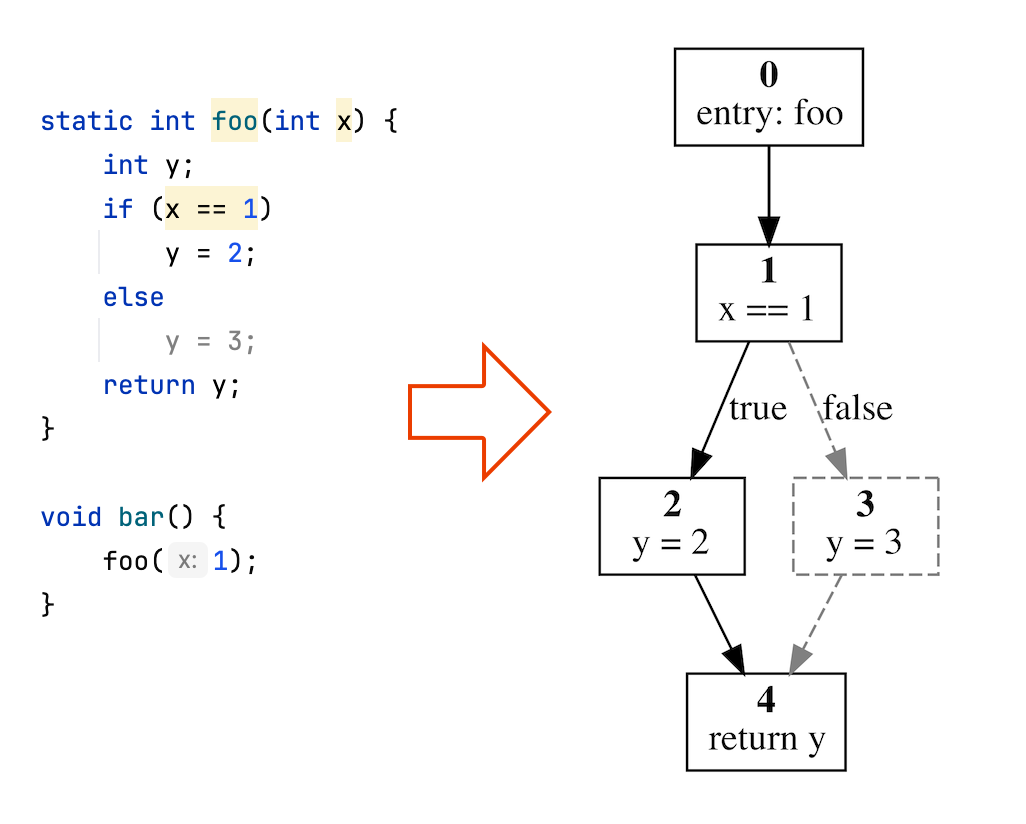CLion builds the corresponding graphs for each function. Each graph has one start node and one exit node, which correspond to the function’s entry and exit. By visiting the nodes of this graph from the start node towards the exit node, CLion can collect some valuable information.

For instance, CLion remembers which values may be stored in each variable for each statement. In the example above, CLion knows that at nodes 0 and 1, the parameter `x` always equals 1. This is because there’s only one call site for the function `foo`, which passes the value 1 in the argument. This being the case, CLion concludes that the condition `x == 1` at node 1 will always be `true`, and so the control flow never goes to node 3. In node 4, the variable y may only hold the value 2, since the control flow may come only from node 2 and never from node 3. Thus, CLion concludes that:

1. Function `foo` always returns the value 2
2. Condition `x == 1` is always `true`
3. Statement `y = 3` is never reachable

Now, let’s look at a more complex example: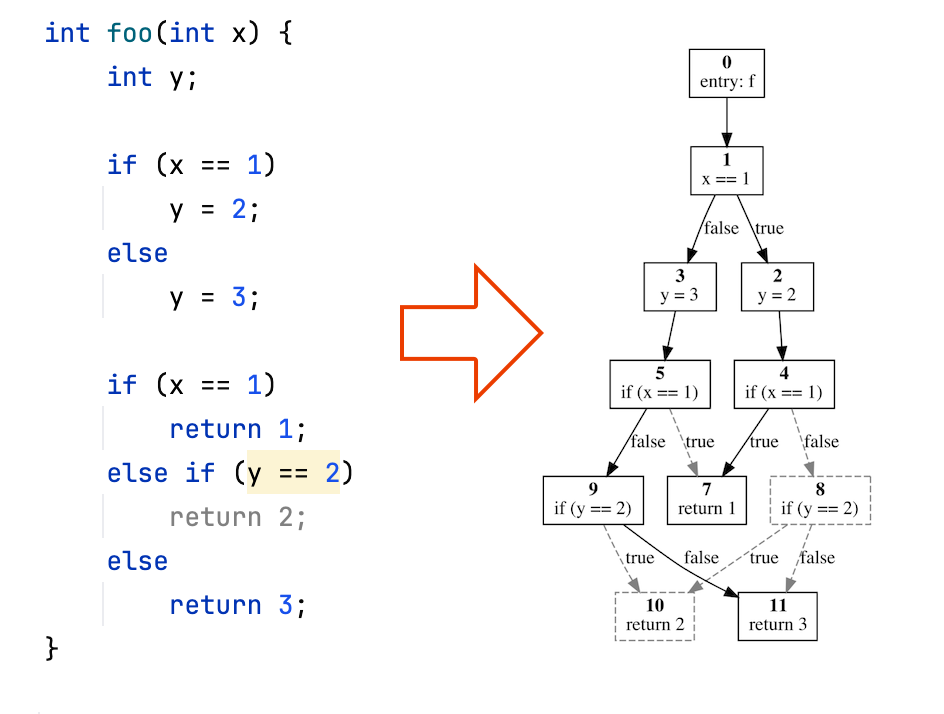Here we have two `if` blocks, and the way the first block is executed influences the functionality of the second block. To support this kind of evaluation, CLion splits the exit statements of the `if` statement into two different contexts: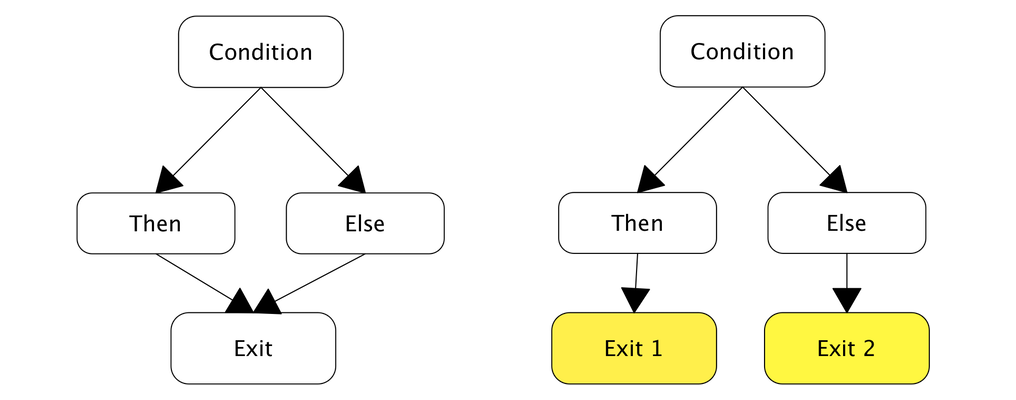The subsequent nodes of the control flow graph are duplicated. They appear twice – one for the `Then` branch of the `if `statement and the second for the `Else` branch. In the first “clone” variable, `x` holds the value 1 (since it corresponds to the positive branch of the` if `statement) and `y` holds the value 2 (which was stored in the node 2). In the second “clone”, `x ! = 1` and `y` is 3.

The second condition `x == 1`, corresponds to the two cloned nodes 4 and 5. In node 4, the condition always holds true, since `x == 1`. Meanwhile, in node 5, it is always false. Hence, nodes 8 and 10 are never reachable, and condition `y == 2` has only one reachable clone – node 9. In this node `y ! = 2`, and hence this condition is always false.

## Data flow analysis in action

Let’s see how these techniques help CLion find subtle bugs in C++ programs! We decided to analyze the Z3 theorem prover, and here are the findings from our data flow analysis in CLion.

Here, the variable `u` is initialized to `null_lpvar` and then possibly reassigned to the same value (because `j == null_lpvar` in the `if` condition). Hence the condition `u == null_lpvar` is always `true`. Since there is a return in the `true` branch of this `if `clause, all subsequent code is marked as non-reachable (reported as #6951):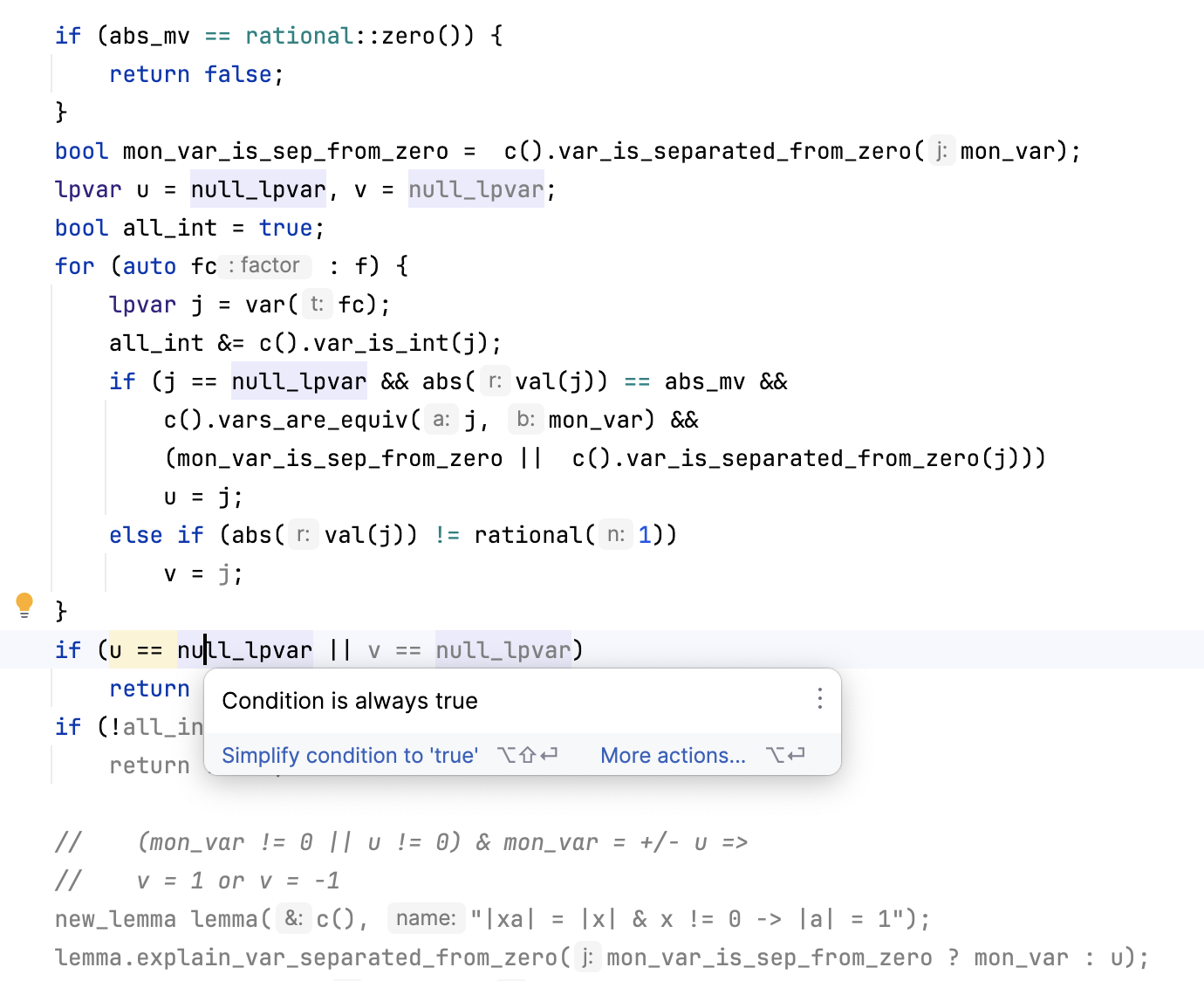Another case can be found below. Here, the unsigned variable `i` is always equal to or greater than zero and, in the `else` branch, it is non zero. Hence, the `i > 0` condition is always true (reported as #6952):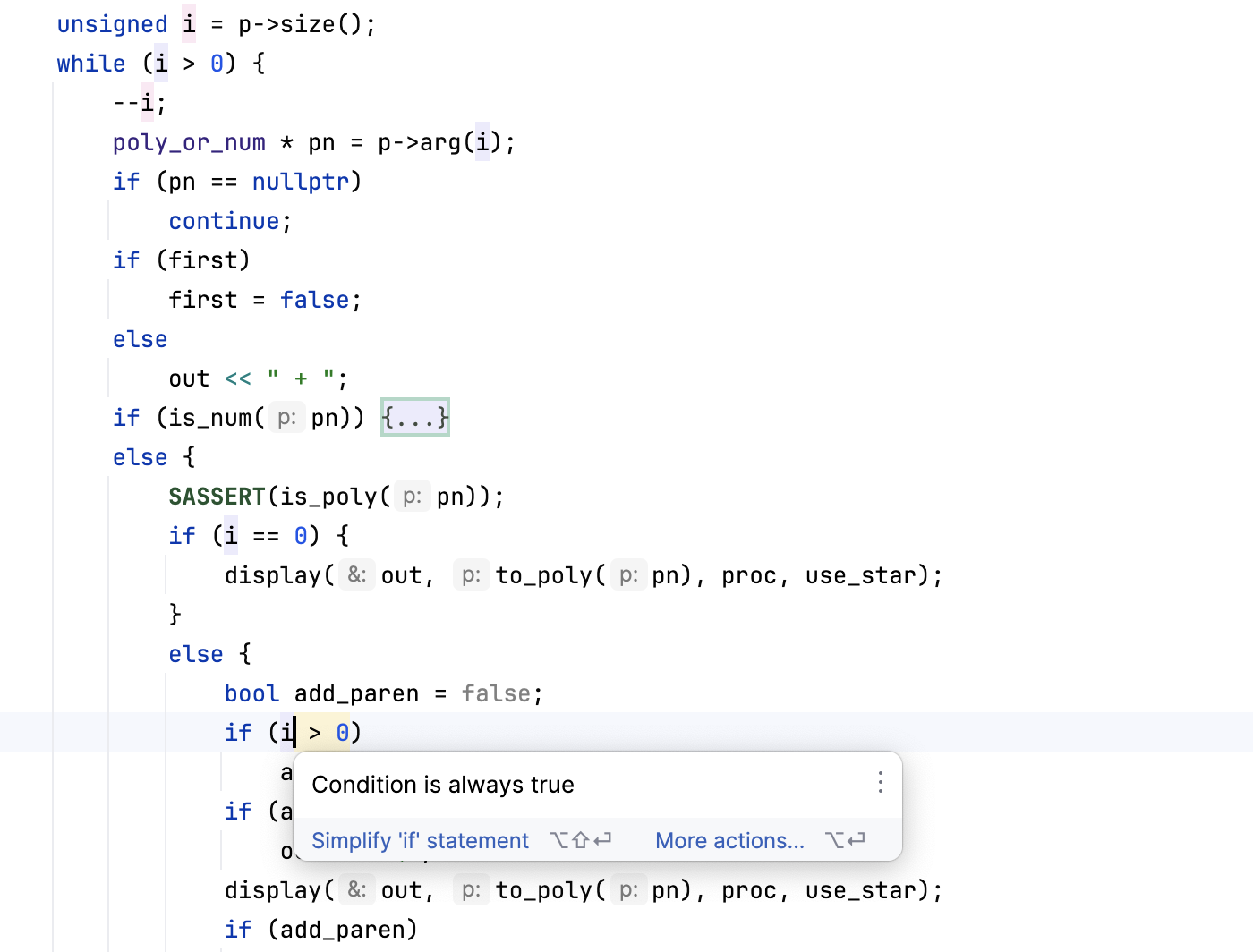In this blog post we have covered one of our dataflow inspections – Constant conditions. There are many other dataflow inspections produced by CLion and we will cover some of them in upcoming blog posts. In which cases do you find code analysis useful? Share your examples with us in the comments below!

## Try out

You can try out these improvements in CLion 2023.3 Release Candidate or in CLion Nova.